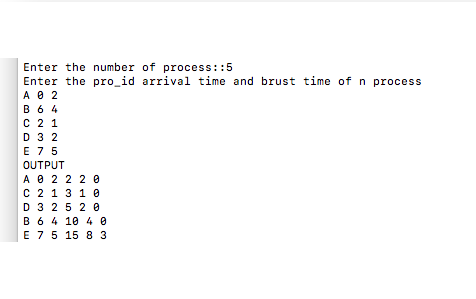Implementations of FCFS scheduling algorithm using C++

In this article, we are going to learn about First Come First Served (FCFC) scheduling algorithm using C++ program.
Submitted by Aleesha Ali, on January 25, 2018

Scheduling

Scheduling can be explained as to schedule a process in CPU(Central Processing Unit), with the help of some algorithms that are given below:

• FCFS
• SJF (Non Preemptive)
• SJF (Preemptive)
• Priority (Non Preemptive)
• Priority (Preemptive)
• Round Robin
• LJF
• HRRN

Non Preemptive: We can not remove a process until it completes it execution.

Preemptive: If a process of higher priority comes then first CPU will be assign to the Process with higher priority first.

Scheduling criteria tells us that any algorithm is how much efficient, the main criteria of scheduling are given below:

• CPU Utilization
• Throughput
• Arrival time
• Turn around time
• Waiting time
• Completion time
• Burst time

*Ready Queue is a queue where all the processes wait to get CPU for its execution.

CPU Utilization: The amount of time CPU is busy.

Throughput: The number of process computed per unit time.

Arrival time: The time at which the process enters into ready queue.

Turn around time: The interval between the time of submission of a process to the time of completion.

Waiting time: The total amount of the time a process spends in ready queue.

Completion time: The time at which process completes its execution.

Burst time: The time needed by CPU to completes its execution.

FIRST COME FIRST SERVED (FCFS) Algorithm

As the name suggests in this algorithm process are schedule according to their arrival time, the process that comes first will be scheduled first and it will be in CPU until it completes it burst time.

C++ Program for FCFS Scheduling

//Implementation fo FIRST COME FIRST SERVE Using C++

#include <iostream>
#include <algorithm>
#include <stdio.h>

using namespace std;

struct Schedule
{
string pro_id;
int artime,bt,ct,ta,wt;
/*
artime = Arrival time,
bt = Burst time,
ct = Completion time,
ta = Turn around time,
wt = Waiting time
*/

};

bool compare(Schedule p1,Schedule p2)
{
return p1.artime<p2.artime;
/* This process will always return TRUE if above condition comes*/
}

int main()
{

Schedule process;
//An array of Processes

int cpunon=0;

int n,i;
//n = number of processes, i= iteration variable

float percentage;

printf("Enter the number of process::");
scanf("%d",&n);

printf("Enter the pro_id arrival time and brust time of n process\n");
for(i=0;i<n;i++)
{
cin>>process[i].pro_id;
scanf("%d",&process[i].artime);
scanf("%d",&process[i].bt);
}

sort(process,process+n,compare);

/*sort is a predefined funcion  defined in algorithm.h header file,
it will sort the processes according to their arrival time*/

cpunon=process.artime-0;

// initial values

process.ct=process.artime+process.bt;
process.ta=process.ct-process.artime;
process.wt=0;

for(i=1;i<n;i++)
{
if(process[i].artime<=process[i-1].ct)
{
process[i].ct=process[i-1].ct+process[i].bt;
process[i].ta=process[i].ct-process[i].artime;
process[i].wt=process[i].ta-process[i].bt;
}
else
{
process[i].ct=process[i].bt+process[i].artime;
process[i].ta=process[i].ct-process[i].artime;
process[i].wt=process[i].ta-process[i].bt;
cpunon+=process[i].artime-process[i-1].ct;
}
}

percentage=(float)((process[n-1].ct-cpunon)/process[n-1].ct)*100;

cout<<"OUTPUT"<<endl;
for(i=0;i<n;i++)
{
//keep all statements in one line
cout<<process[i].pro_id<<" "<<process[i].artime<<" "
<<process[i].bt<<" "<<process[i].ct<<" "
<<process[i].ta<<" "<<process[i].wt;

cout<<endl;
}

return 0;
}

OutputPreparation